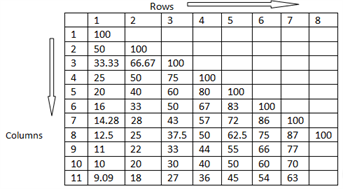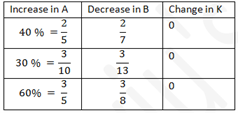# Percentage Equivalence Table

As the CAT exam is all about speed and efficiency, the candidates are required to be able to solve the questions effectively in the least possible time. For that, knowing various methodologies and techniques is necessary and one of such important topic is the percentage equivalence table.

In order to solve different questions, it is always good to know the reciprocals of numbers till 20. Once the reciprocals are known, the value of several fractions can be easily obtained and the related questions can be solved quickly and effectively. The table, is shown below along with illustrations.

#### The Percentage Equivalence Table is read as follows:First row, first column➜ 1/1 x 100= 100%

Second row, first column➜ 1/2 x 100= 50%

Fourth row, Third column➜ 3/4 x 100= 75%

It is advantageous to know the reciprocals of prime numbers (the reciprocals below are multiplied by 100)

1/13= 7.69 1/17= 5.88 1/19= 5.26 1/23= 4.35 1/29= 3.45

### Application of the Percentage Equivalence Table: Examples

Example 1: Suppose you need to do the approximate multiplication 42 x 142

Solution:

The faster way to do it is to take 42 x 142 as 42 x 14.2 x 10 = 42 × 17 × 100 × 10 (as 14.28 = 1/7×100)

Thus 42 × 142= 6000.

Example 2:  Similarly for the multiplication 114 x 8.37 = 114 × 5/6 ×10 ( 83= 5/6×100) = 950 (Approximately)

Initially, it may take time to get used to the conversion, but after repeated practice, it saves a lot of time for calculation and can be one of your best time-saving strategies for the exam.Stay tuned with Byju’s to get various lessons and video lectures on different CAT exam topics along with the latest CAT syllabus and CAT Question Paper. The candidates are also provided with the detailed section-wise explanation and various solved questions for effective preparation.